# 1.17. 神经网络模型（有监督）

## 1.17.1. 多层感知器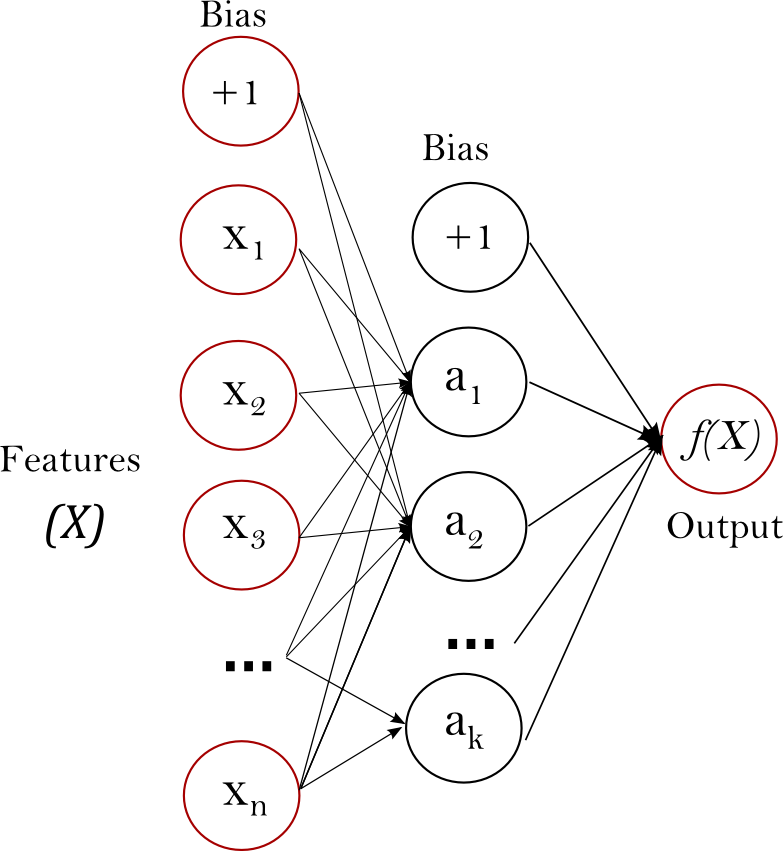• 可以学习得到非线性模型。
• 使用partial_fit 可以学习得到实时模型(在线学习)。

• 具有隐藏层的 MLP 具有非凸的损失函数，它有不止一个的局部最小值。 因此不同的随机权重初始化会导致不同的验证集准确率。
• MLP 需要调试一些超参数，例如隐藏层神经元的数量、层数和迭代轮数。
• MLP 对特征归一化很敏感.

## 1.17.2. 分类

MLPClassifier 类实现了通过 Backpropagation 进行训练的多层感知器（MLP）算法。

MLP 在两个 array 上进行训练:大小为 (n_samples, n_features) 的 array X 储存表示训练样本的浮点型特征向量; 大小为 (n_samples,) 的 array y 储存训练样本的目标值（类别标签）:

>>> from sklearn.neural_network import MLPClassifier
>>> X = [[0., 0.], [1., 1.]]
>>> y = [0, 1]
>>> clf = MLPClassifier(solver='lbfgs', alpha=1e-5,
...                     hidden_layer_sizes=(5, 2), random_state=1)
...
>>> clf.fit(X, y)
MLPClassifier(activation='relu', alpha=1e-05, batch_size='auto',
beta_1=0.9, beta_2=0.999, early_stopping=False,
epsilon=1e-08, hidden_layer_sizes=(5, 2),
learning_rate='constant', learning_rate_init=0.001,
max_iter=200, momentum=0.9, n_iter_no_change=10,
nesterovs_momentum=True, power_t=0.5, random_state=1,
shuffle=True, solver='lbfgs', tol=0.0001,
validation_fraction=0.1, verbose=False, warm_start=False)


>>> clf.predict([[2., 2.], [-1., -2.]])
array([1, 0])



MLP 可以为训练数据拟合一个非线性模型。 clf.coefs_ 包含了构建模型的权值矩阵:

>>> [coef.shape for coef in clf.coefs_]
[(2, 5), (5, 2), (2, 1)]



MLP 算法使用的是反向传播的方式。 更准确地说，它使用了通过反向传播计算得到的梯度和某种形式的梯度下降来进行训练。 对于分类来说，它最小化交叉熵损失函数，为每个样本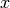给出一个向量形式的概率估计>>> clf.predict_proba([[2., 2.], [1., 2.]])
array([[  1.967...e-04,   9.998...-01],
[  1.967...e-04,   9.998...-01]])



MLPClassifier 通过应用 Softmax 作为输出函数来支持多分类。

>>> X = [[0., 0.], [1., 1.]]
>>> y = [[0, 1], [1, 1]]
>>> clf = MLPClassifier(solver='lbfgs', alpha=1e-5,
...                     hidden_layer_sizes=(15,), random_state=1)
...
>>> clf.fit(X, y)
MLPClassifier(activation='relu', alpha=1e-05, batch_size='auto',
beta_1=0.9, beta_2=0.999, early_stopping=False,
epsilon=1e-08, hidden_layer_sizes=(15,),
learning_rate='constant', learning_rate_init=0.001,
max_iter=200, momentum=0.9, n_iter_no_change=10,
nesterovs_momentum=True, power_t=0.5,  random_state=1,
shuffle=True, solver='lbfgs', tol=0.0001,
validation_fraction=0.1, verbose=False, warm_start=False)
>>> clf.predict([[1., 2.]])
array([[1, 1]])
>>> clf.predict([[0., 0.]])
array([[0, 1]])



## 1.17.3. 回归

MLPRegressor 类多层感知器（MLP）的实现，在使用反向传播进行训练时的输出层没有使用激活函数，也可以看作是使用恒等函数（identity function）作为激活函数。 因此，它使用平方误差作为损失函数，输出是一组连续值。

MLPRegressor 还支持多输出回归，其中一个样本可以有多个目标值。

## 1.17.4. 正则化

MLPRegressor 类和 MLPClassifier 类都使用参数 alpha 作为正则化( L2 正则化)系数，正则化通过惩罚大数量级的权重值以避免过拟合问题。 下面的图表展示了不同的 alpha 值下的决策函数的变化。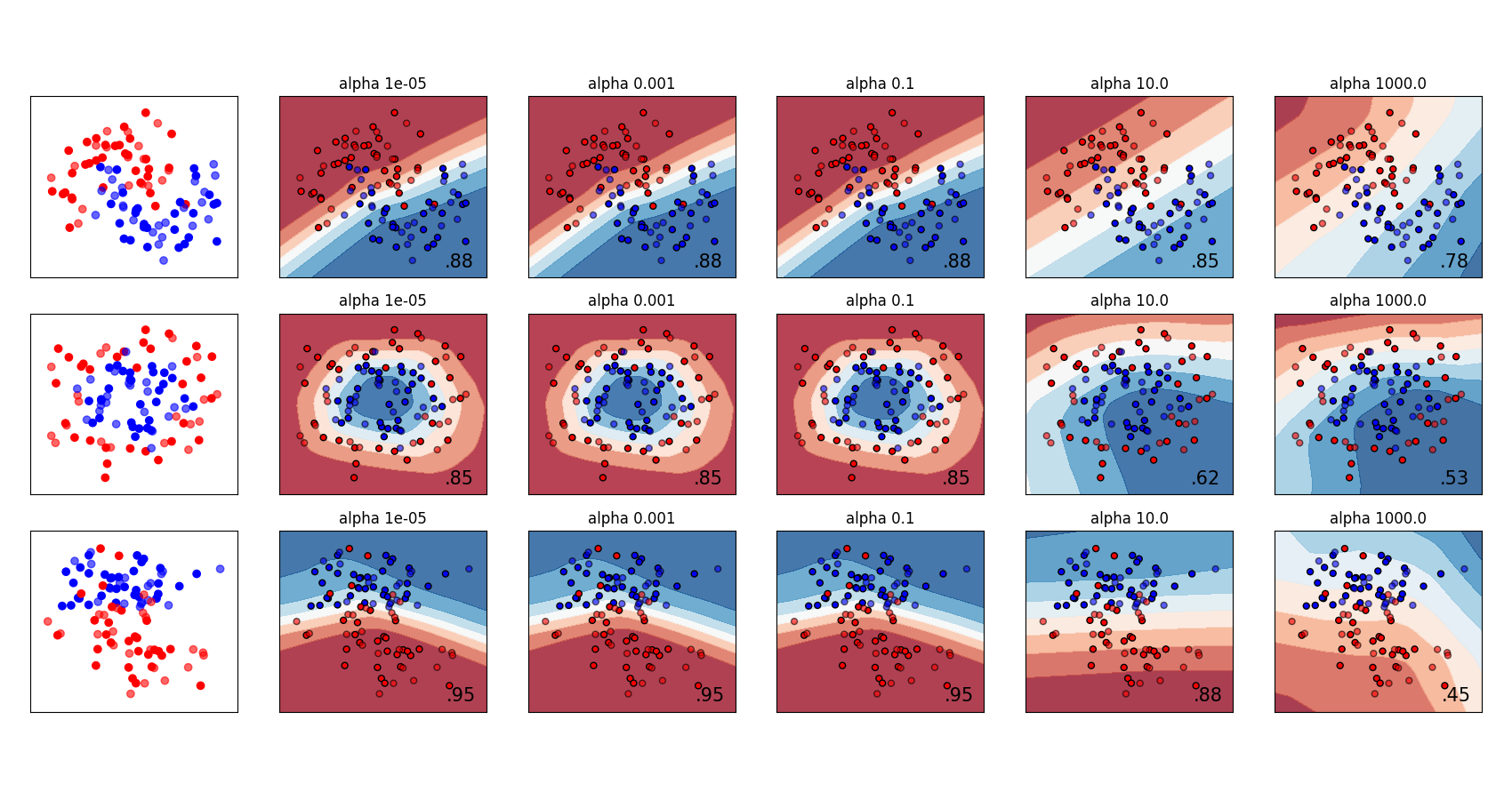## 1.17.5. 算法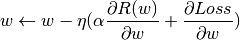Adam 类似于 SGD，因为它是 stochastic optimizer （随机优化器），但它可以根据低阶矩的自适应估计自动调整参数更新的量。

L-BFGS 是利用 Hessian 矩阵来近似函数的二阶偏导数的求解器，它使用 Hessian 的逆矩阵来近似进行参数更新。 该实现使用 Scipy 版本的 L-BFGS

## 1.17.7. 数学公式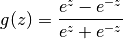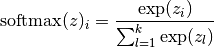MLP 根据特定问题使用不同的损失函数。 二分类问题的损失函数的是交叉熵，具体形式如下，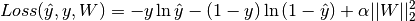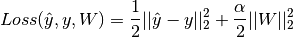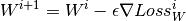## 1.17.8. 实用技巧

• 多层感知器对特征的缩放是敏感的，所以它强烈建议您归一化你的数据。 例如，将输入向量 X 的每个属性放缩到到 [0, 1] 或 [-1，+1] ，或者将其标准化使它具有 0 均值和方差 1。注意，为了得到有意义的结果，您必须对测试集也应用 相同的 尺度缩放。 您可以使用 StandardScaler 进行标准化。

py from sklearn.preprocessing import StandardScaler scaler = StandardScaler() # Don't cheat - fit only on training data scaler.fit(X_train) X_train = scaler.transform(X_train) # apply same transformation to test data X_test = scaler.transform(X_test) 另一个推荐的方法是在 Pipeline 中使用的 StandardScaler 。 * 最好使用 GridSearchCV 找到一个合理的正则化参数，通常范围是在 10.0 ** -np.arange(1, 7) 。 * 据经验可知，我们观察到 L-BFGS 收敛速度是更快的并且是小数据集上更好的解决方案。对于规模相对比较大的数据集，Adam 是非常鲁棒的。 它通常会迅速收敛，并得到相当不错的表现。另一方面，如果学习率调整得正确， 使用 momentum 或 nesterov’s momentum 的 SGD 可以比这两种算法更好。

## 1.17.9. 使用 warm_start 的更多控制

>>> X = [[0., 0.], [1., 1.]]
>>> y = [0, 1]
>>> clf = MLPClassifier(hidden_layer_sizes=(15,), random_state=1, max_iter=1, warm_start=True)
>>> for i in range(10):
...     clf.fit(X, y)
...     # additional monitoring / inspection
MLPClassifier(...